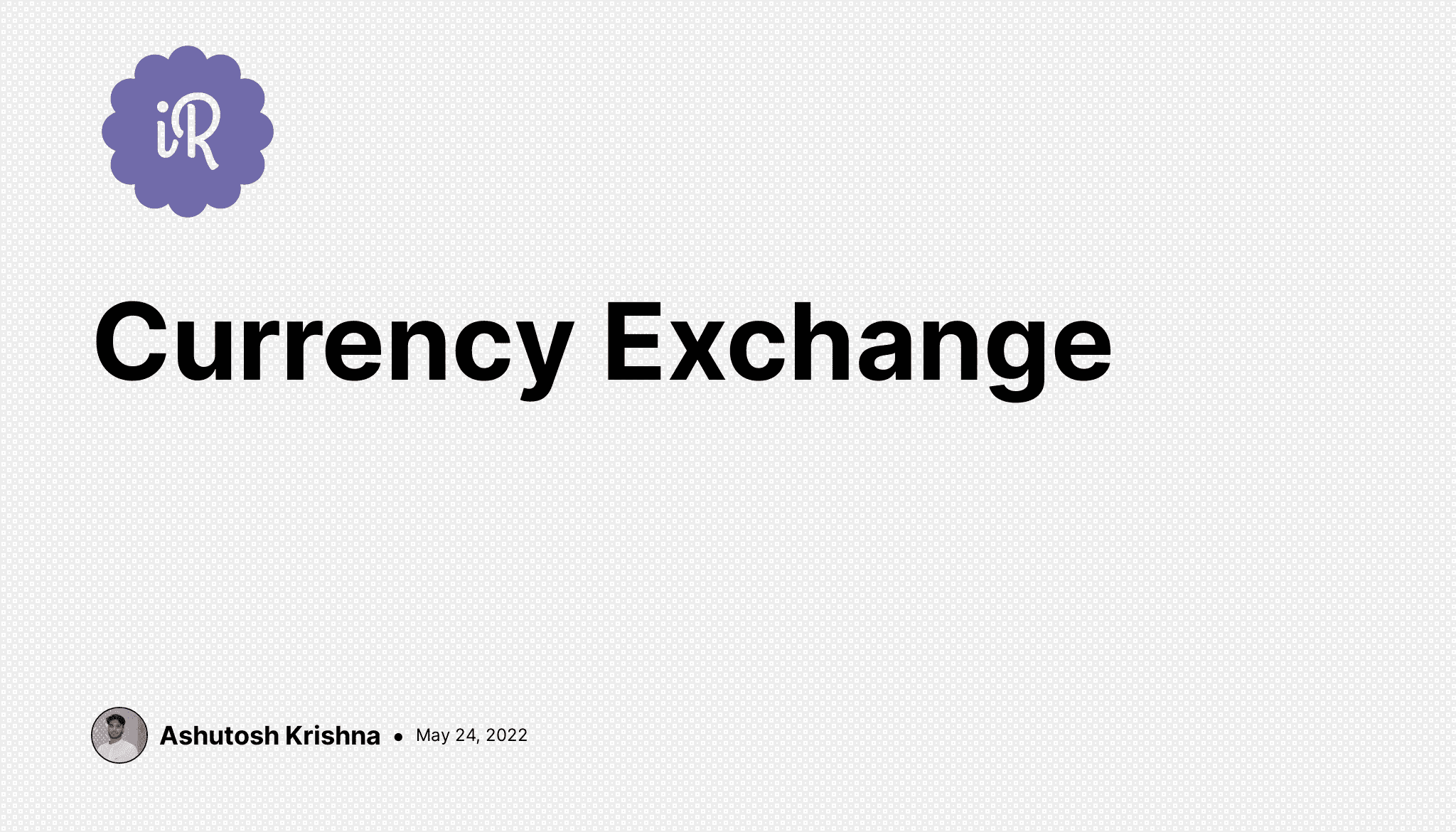# Ashutosh Writes# Currency Exchange

Instructions

Your friend Chandler plans to visit exotic countries all around the world. Sadly, Chandler's math skills aren't good. He's pretty worried about being scammed by currency exchanges during his trip - and he wants you to make a currency calculator for him. Here are his specifications for the app:

#### 1. Estimate value after exchange

Create the `exchange_money()` function, taking 2 parameters:

1. `budget` : The amount of money you are planning to exchange.
2. `exchange_rate` : The amount of domestic currency equal to one unit of foreign currency.

This function should return the value of the exchanged currency.

Note: If your currency is USD and you want to exchange USD for EUR with an exchange rate of `1.20`, then `1.20 USD == 1 EUR`.

``````>>> exchange_money(127.5, 1.2)
106.25
``````

#### 2. Calculate currency left after an exchange

Create the `get_change()` function, taking 2 parameters:

1. `budget` : Amount of money before exchange.
2. `exchanging_value` : Amount of money that is taken from the budget to be exchanged.

This function should return the amount of money that is left from the budget.

``````>>> get_change(127.5, 120)
7.5
``````

#### 3. Calculate value of bills

Create the `get_value_of_bills()` function, taking 2 parameters:

1. `denomination` : The value of a single bill.
2. `number_of_bills` : Amount of bills you received.

This exchanging booth only deals in cash of certain increments. The total you receive must be divisible by the value of one "bill" or unit, which can leave behind a fraction or remainder. Your function should return only the total value of the bills (excluding fractional amounts) the booth would give back. Unfortunately, the booth gets to keep the remainder/change as an added bonus.

``````>>> get_value_of_bills(5, 128)
640
``````

#### 4. Calculate number of bills

Create the `get_number_of_bills()` function, taking `budget` and `denomination`.

This function should return the number of new currency bills that you can receive within the given budget. In other words: How many whole bills of new currency fit into the amount of old currency you have in your budget? Remember -- you can only receive whole bills, not fractions of bills, so remember to divide accordingly. Effectively, you are rounding down to the nearest whole bill/denomination.

``````>>> get_number_of_bills(127.5, 5)
25
``````

#### 5. Calculate value after exchange

Create the `exchangeable_value()` function, taking `budget`, `exchange_rate`, `spread`, and `denomination`.

Parameter `spread` is the percentage taken as an exchange fee, written as an integer. It needs to be converted to decimal by dividing it by 100. If `1.00 EUR == 1.20 USD` and the spread is `10`, the actual exchange rate will be: `1.00 EUR == 1.32 USD` because 10% of 1.20 is 0.12, and this additional fee is added to the exchange.

This function should return the maximum value of the new currency after calculating the exchange rate plus the spread. Remember that the currency denomination is a whole number, and cannot be sub-divided.

Note: Returned value should be `int` type.

``````>>> exchangeable_value(127.25, 1.20, 10, 20)
80
>>> exchangeable_value(127.25, 1.20, 10, 5)
95
``````

#### 6. Calculate non-exchangeable value

Create the `non_exchangeable_value()` function, taking `budget`, `exchange_rate`, `spread`, and `denomination`.

This function should return the value that is not exchangeable due to the denomination of the bills. Remember - this booth gets to keep the change in addition to charging an exchange fee. Start by calculating the value you would receive if you were able to keep subdivided bills, then subtract the amount you would receive in whole bills. Both amounts should take the spread, or the exchange fee into account.

Note: Returned value should be `int` type.

``````>>> non_exchangeable_value(127.25, 1.20, 10, 20)
16
>>> non_exchangeable_value(127.25, 1.20, 10, 5)
1
``````

## Solution

``````def exchange_money(budget, exchange_rate):
"""

:param budget: float - amount of money you are planning to exchange.
:param exchange_rate: float - unit value of the foreign currency.
:return: float - exchanged value of the foreign currency you can receive.
"""

return budget / exchange_rate

def get_change(budget, exchanging_value):
"""

:param budget: float - amount of money you own.
:param exchanging_value: int - amount of your money you want to exchange now.
:return: float - amount left of your starting currency after exchanging.
"""

return budget - exchanging_value

def get_value_of_bills(denomination, number_of_bills):
"""

:param denomination: int - the value of a bill.
:param number_of_bills: int - amount of bills you received.
:return: int - total value of bills you now have.
"""

return denomination * number_of_bills

def get_number_of_bills(budget, denomination):
"""

:param budget: float - the amount of money you are planning to exchange.
:param denomination: int - the value of a single bill.
:return: int - number of bills after exchanging all your money.
"""

return budget // denomination

"""

:param budget: float - the amount of your money you are planning to exchange.
:param exchange_rate: float - the unit value of the foreign currency.
:param spread: int - percentage that is taken as an exchange fee.
:param denomination: int - the value of a single bill.
:return: int - maximum value you can get.
"""
new_exchange_rate = exchange_rate * (1 + spread/100)
exchange_value = exchange_money(budget, new_exchange_rate)
number_of_bills = get_number_of_bills(exchange_value, denomination)

return get_value_of_bills(denomination, number_of_bills)

"""

:param budget: float - the amount of your money you are planning to exchange.
:param exchange_rate: float - the unit value of the foreign currency.
:param spread: int - percentage that is taken as an exchange fee.
:param denomination: int - the value of a single bill.
:return: int non-exchangeable value.
"""

new_exchange_rate = exchange_rate * (1 + spread/100)
exchange_value = exchange_money(budget, new_exchange_rate)

return int(exchange_value % denomination)
``````